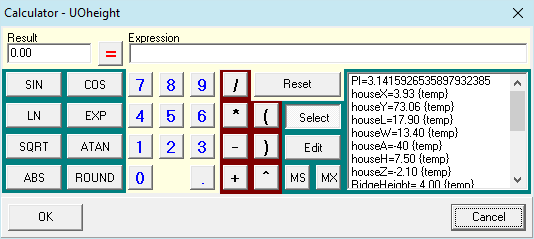# P&K KFA

## CalculatorUniversal calculator, which can be invoked in input fields and table cells with the right mouse button, if defined as real.

In Expression, one can write a formula, which can be solved with the key =. The result will be stored in the entry field Result. The value of Result can be returned with OK (OK doesn't calculate). Besides below stated Mnemonics, owner definable constants and functions are allowed. If undefined variables are encountered, the user will be prompted for their value (e.g. Tq and R). Real variables defined in the form in which the calculator was invoked, are also available. They are marked with an {temp}. In case of a table, the Real variables found in the clicked row are available. Comments can be added with a leading blank.

 Key Caption Function Description ^ basex Returns base raised X. Only positive numbers are allowed for base! ( ) Brackets for Expressions and Functions. LN Ln(x) Returns the natural logarithm of a numeric expression. ABS Abs(x) Returns the absolute value of a number. COS Cos(x) Returns the cosine of a specified angle. ATAN ArcTan(x) Returns the arctangent of a specified numeric expression. SIN Sin(x) Returns the sine of a specified angle. SQRT Sqr(x) Returns the square root of the argument X. ROUND Round (x) Rounds a Real to the nearest Integer value. EXP Exp(x) Returns e raised to a specified power, where e is the base of natural logarithms. Select Select Constant or Function. Toggles the List into Select-Mode. Edit Edit Constant or Function. Toggles the List into Edit-Mode. MS Stores the current Result in the constant MEM. The name can be modified in Edit. MX Stores the Expression. Reset Clears Expression and Result.

Language: